sbi clerk :: numerical ability :: test 7

Home sbi clerk / numerical ability Questions and Answers

1.  Directions(Q.1 to Q.5 ):
Line chart given below shows number of labors (men and women) working in six different years. Study the data carefully and answer the following questions.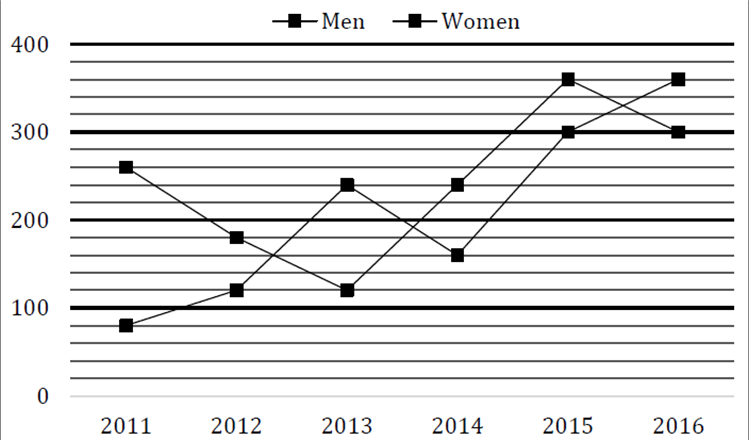Total number of Men working in 2012 and 2013 together is what percent of the total number of labors (Men + Women) working in 2014?

a

60%

b

70%

c

80%

d

90%

e

40%View AnswerDiscuss
 Answer : Option D Explanation : Required % = $\frac{{120 + 240}}{{160 + 240}} \times 100 = \frac{{360}}{{400}} \times 100 = 90{\rm{\% }}$

2.  Average number of Women working in 2014, 2015 and 2016 together is how much more/less than average number of Men working in 2011, 2014 and 2016 together?

a

100

b

80

c

90

d

70

e

None of the given optionsView AnswerDiscuss
 Answer : Option A Explanation : Average number of Women working in 2014, 2015 and 2016 together = $\frac{1}{3}\left[ {240 + 360 + 300} \right] = \frac{{900}}{3} = 300$ Average number of Men working in 2011, 2014 and 2016 together = $\frac{1}{3}\left[ {80 + 160 + 360} \right]$ $= \frac{{600}}{3} = 200$ Required difference = 300−200 = 100

3.  Number of Men working in 2017 is 15% more than that of 2015 while number of Women working in 2017 is 40% less than that of 2014. Find total number of labors (Men + Women) working in 2017?

a

561

b

456

c

489

d

594

e

630View AnswerDiscuss
 Answer : Option C Explanation : Number of Men working in 2017 $= \frac{{115}}{{100}} \times 300 = 345$ Number of Women working in 2017 $= \frac{{60}}{{100}} \times 240 = 144$ Total number of labors working in 2017 = 345+144=489

4.  Find the ratio between total number of Labors working in 2012 and 2013 together to total number of labors working in 2015 and 2016 together?

a

2 : 1

b

1 : 2

c

35 : 66

d

11 : 10

e

None of the given optionsView AnswerDiscuss
 Answer : Option B Explanation : Required Ratio = $\frac{{\left( {120 + 180} \right) + \left( {240 + 120} \right)}}{{\left( {300 + 360} \right) + \left( {360 + 300} \right)}}$ $= \frac{{300 + 360}}{{660 + 660}} = \frac{{660}}{{1320}} = \frac{1}{2}$

5.  Total number of Men working in all six years is how much more/less than total number of Women working in all six years together?

a

None of the given options

b

140

c

160

d

180

e

200View AnswerDiscuss
 Answer : Option E Explanation : Total number of Men working in all six years = 80+120+240+160+300+360=1260 Total number of Women working in all six years = 260+180+120+240+360+300=1460 Required difference = 1460−1260=200

6.  Directions(Q.6 to Q.10 ):
Find the wrong number in the following number series:

4, 5.1, 7.3, 10.6, 15, 20, 27.1

a

5.1

b

4

c

7.3

d

20

e

27.1View AnswerDiscuss
 Answer : Option D Explanation : /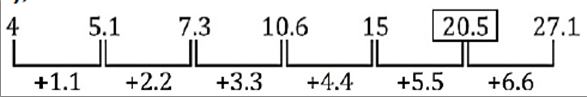7.  2, 3, 8, 31, 154, 924, 6460

a

924

b

6460

c

154

d

8

e

31View AnswerDiscuss
 Answer : Option A Explanation : /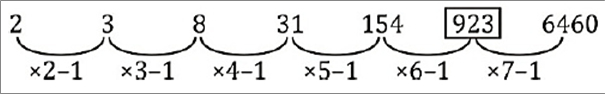8.  251, 252, 254, 227, 243, 118, 154

a

251

b

252

c

227

d

243

e

154View AnswerDiscuss
 Answer : Option B Explanation : /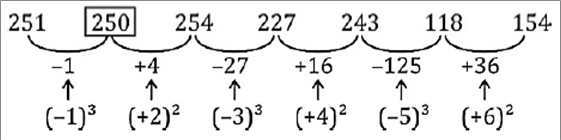9.  141, 156, 147, 162, 153, 165, 159

a

156

b

153

c

147

d

165

e

159View AnswerDiscuss
 Answer : Option D Explanation : /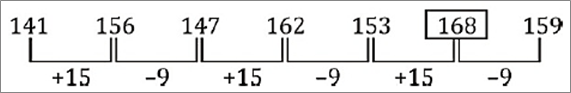10.  2, 6, 10, 19, 36, 69, 134

a

134

b

69

c

6

d

2

e

10View AnswerDiscuss
 Answer : Option C Explanation : /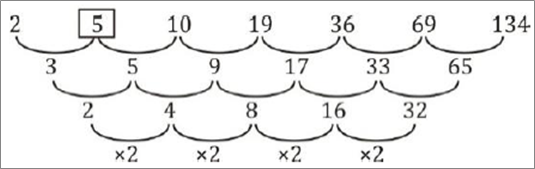11.  Directions(Q.11 to Q.21 ):

0.5, 2, 1, 4, 32, 512, 16384

a

1

b

2

c

4

d

32

e

512View AnswerDiscuss
 Answer : Option B Explanation : /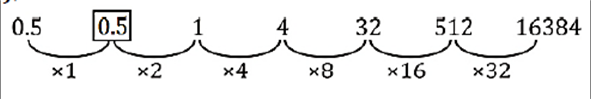12.  Ratio of present ages of A and B is 16:7. After 12 years, A’s age is twice of B’s age then find present ages of A and B?

a

64yr; 28yr

b

80yr; 35yr

c

None of these

d

96yr; 42 yr

e

102yr; 49yrView AnswerDiscuss
 Answer : Option D Explanation : Let present age of A and B be 16x yr and 7x yr respectively ATQ $\frac{{16x + 12}}{{7x + 12}} = \frac{2}{1}$ ⇒2x=12 ⇒x=6 Present age of A=96 yr Present age of B= 42 yr

13.  A man invested a certain sum in scheme A at 15% p.a. for 2 years and earned Rs 1950 as simple interest. He increased his sum by Rs. ’x’ and invested in another scheme B at 10% p.a. C.I. for 2 years and received Rs. 1680 as compound interest. Find the value of ’x’ ?

a

Rs. 1750

b

Rs. 1500

c

Rs. 1250

d

None of these

e

Rs. 1850View AnswerDiscuss
 Answer : Option B Explanation : Sum= $\frac{{1950 \times 100}}{{2 \times 15}} = {\rm{Rs\;}}6500$ CI in 2 years at 10% per annum = 10+10+$\frac{{10 \times 10}}{{100}}$ =21% ATQ (6500+x)× $\frac{{21}}{{100}}$ =1680 ⇒(6500+x)=8000 x=Rs 1500

14.  In a class there are 30 girls and 15 boys and total average weight of class is 47$\frac{7}{{15}}$ kg. Total average weight of boys is 58 kg. Find the approximate average weight of girls ?

a

32 kg

b

42 kg

c

52 kg

d

35 kg

e

50 kgView AnswerDiscuss
 Answer : Option B Explanation : Total weight of students = $47\frac{7}{{15}}\left( {15 + 30} \right)$ = 2136 kg Total weight of boys = 15× 58 = 870 kg Average weight of girls = $\left( {\frac{{2136 - 870}}{{30}}} \right){\rm{kg}} = 42.2{\rm{kg}}$ ≃ 42 kg

15.  Ram bought a bike at 20% discount on MRP. After 1 year Ram sell the bike to Ramesh at 10% loss. After 1 year more Ramesh sell the bike at 20% profit to Ranjan. If Ranjan paid Rs. 1,29,600, then find the M.R.P. of the bike ?

a

1,50,000

b

2,25,000

c

1,40,000

d

2,00,000

e

1,80,000View AnswerDiscuss
 Answer : Option A Explanation : Ram’s cost price = M.R.P. × $\frac{{80}}{{100}}$ Ramesh C.P = M.R.P. × $\frac{{80}}{{100}} \times \frac{{90}}{{100}}$ Ranjan C.P. = M.R.P. ×$\frac{{80}}{{100}} \times \frac{{90}}{{100}} \times \frac{{120}}{{100}}$ =1,29,600 ⇒ M.R.P. = Rs. 1,50,000